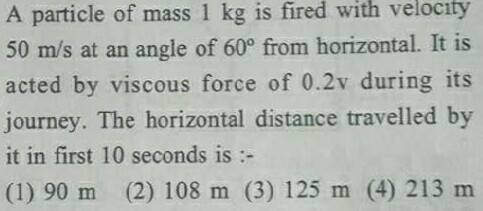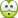# Is there a quicker way to solve this projectile problem?

• Jahnavi

## Homework Statement## The Attempt at a Solution

I solved this problem and got the correct answer 108 m . But I had to solve a DE . This question is from a test where we get around 1 minute to solve a problem . I took half an hour to solve it.

It looks like a subjective type problem .

I am just curious as to whether there is a quicker/smarter way to find one of the options .

#### Attachments

What if you considered just the horizontal motion and the viscous force acting on the horizontal component of the velocity? That way, it still needs a differential equation, but only in one dimension. ## \\ ## It's a very simple D.E. that takes at most 2 minutes to solve for ## v(t) ## and then you can integrate ## v(t) ## from ## t=0 ## to ## t=10 ##.## \\ ## Editing: Yes, I get ## s=108 ## m also. ## \\ ## And note: They make it easy by making ## m=1 \, kg ##, so that ## a=\frac{dv}{dt}=\frac{F}{m}=\frac{-.2v}{1}=-.2 v ##. ## \\ ## Question: Is this the D.E. that you used, or did you try to include the vertical motion?

Last edited:
•Jahnavi and cnh1995
What if you considered just the horizontal motion and the viscous force acting on the horizontal component of the velocity? That way, it still needs a differential equation, but only in one dimension.

I did the sameIs this the D.E. that you used,

Yes !

•I did the sameYes !
The first time you see that D.E. it might take 15 minutes or more to solve, but that is one that I think I have seen hundreds of times, and it is a simple matter to write: ## \frac{dv}{v}=-\lambda \, dt ## ==>> ## \ln| v|=-\lambda t +C ## so that ## v(t)=v_o e^{- \lambda t} ##. The first several times it probably took me a few minutes or longer...

Last edited:
•Jahnavi
It's a very simple D.E. that takes at most 2 minutes

The first time you see that D.E. it might take 15 minutes or more to solve

I can see , you are trying to make me feel better.

Nevertheless , my intent of posting this question was to know if some of the options could be eliminated and correct answer could be chosen without employing calculus .

Nevertheless , my intent of posting this question was to know if some of the options could be eliminated and correct answer could be chosen without employing calculus .
I don't think there is any way besides calculus, but the calculus solution, including the solution of the D.E., and integrating ## v(t) ## is very quick=quick enough that if you spent a minute or two looking for something simpler, I think most likely you would come up empty. I don't see a simpler solution...

•Jahnavi
What if you considered just the horizontal motion and the viscous force acting on the horizontal component of the velocity?
Fine, but you first have to know or discover that it is ok to treat motion components separately for linear drag. For any other function of velocity, the drag due to the overall motion has to be found, then the component of interest taken.

•••